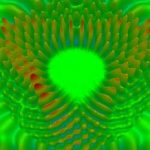Forum Options

Home Forums KNOWLEDGE Gaussian Pulse Description [FDTD]# Gaussian Pulse Description [FDTD]

Question:

I want to setup a Gaussian Modulated Continuous Wave input field for my structure. I have a rough idea what the time offset and half width mean, but is there any documentation on the exact meaning of these variables?

### Responses (9):

• #10213Damian Marek
Participant

Question:

I want to setup a Gaussian Modulated Continuous Wave input field for my structure. I have a rough idea what the time offset and half width mean, but is there any documentation on the exact meaning of these variables?

• #13045Serena Zhang
Participant

Hello, Damian

I went over the tutorial in the attachment and have some questions regarding the formulas on page 5. How did you come up with the formula to calculate the center wavelength if the start and end wavelengths are known? BTW, there is a typo in the formula.

Thanks

Serena

• #13126Damian Marek
Participant

Hi Serena,

The correct formula should be lambda0 = lambda_s + 1/e * (lambda_e – lambda_s)

Thanks for pointing this out.

+1
• #26658Haya AlSharif
Participant

Thank you for the very helpful information.
What is e in the corrected equation of the center wavelength??
lambda0 = lambda_s + 1/e * (lambda_e – lambda_s)

• #26674Damian Marek
Participant

That’s the base of the natural logarithm or Euler’s number with the value: ~2.718

+1
• #25779shengsheng
Participant

I use the formula in the attachment. And my start wavelength is 0.7 um and end wavelength is 2.5 um. I use these data to calculate the time offset and time domain half width. However, I cannot get the imagine as shown in the attachment. Can you give me some suggestions?

• #25847Damian Marek
Participant

You will notice one of the assumptions in the formula on the page containing IV is that the effective bandwidth (wavelength bandwidth divided by center wavelength) needs to be less than 100%. In your case (0.7-2.5)/1.55 is 116% which is greater. So that little helpful equation won’t help as it should.

Try increasing the time delay by an additional 0.5eE-14 s, from what I can tell that should look better.

• #29845amin moazami
Participant

hi , is there any exact formula for getting these two values (time offset & half width)?
for wave lenght = 500 um , i can not find the suitable value ;(

another Q is that , does the mesh size affect on time offset and half width ?

• #43205asmita
Participant

I’d not understand, how to choose the number of runs. As stated in “Deep understanding…”, run should make the GMCW pulse convergent to zero, but what should be the location of the observation point for this. For instance, if I have a waveguide coupled to reonator, then at different points the GMCW pulse will reach at different points. So, how one should decide the total number of time steps for the run?

• #10214Damian Marek
Participant